Finding equation of tangent/normal when point and curve is given

Chapter 6 Class 12 Application of Derivatives
Concept wise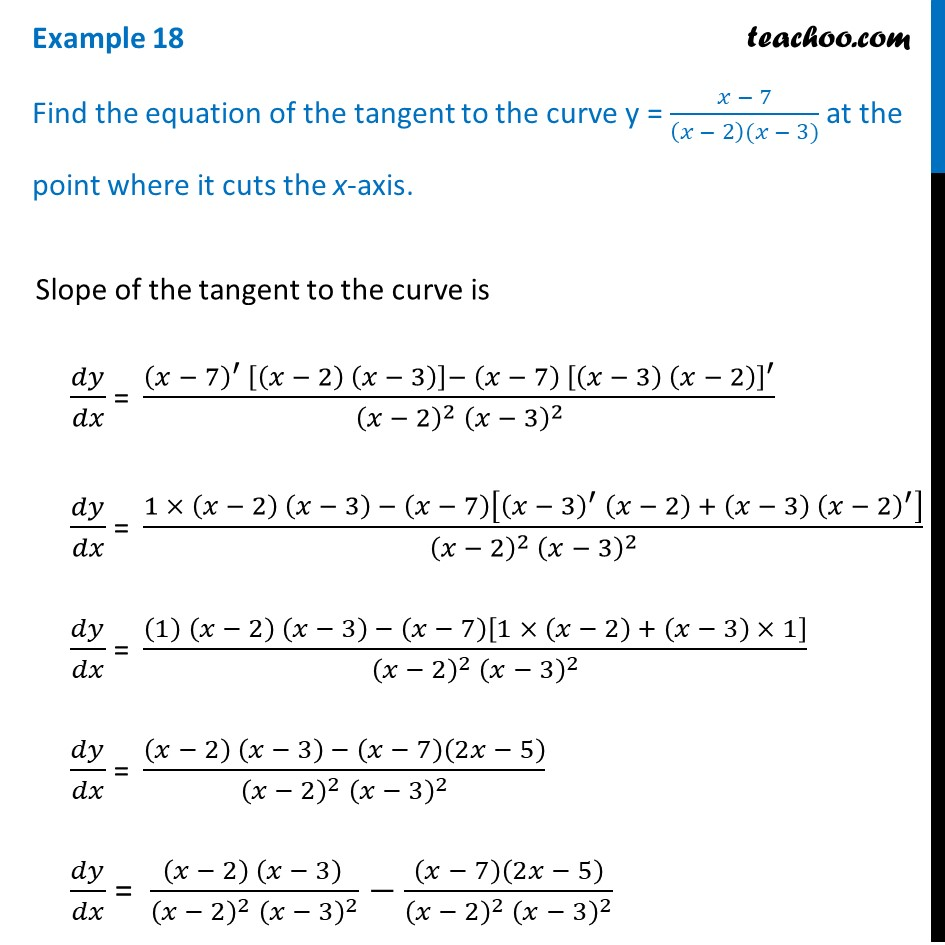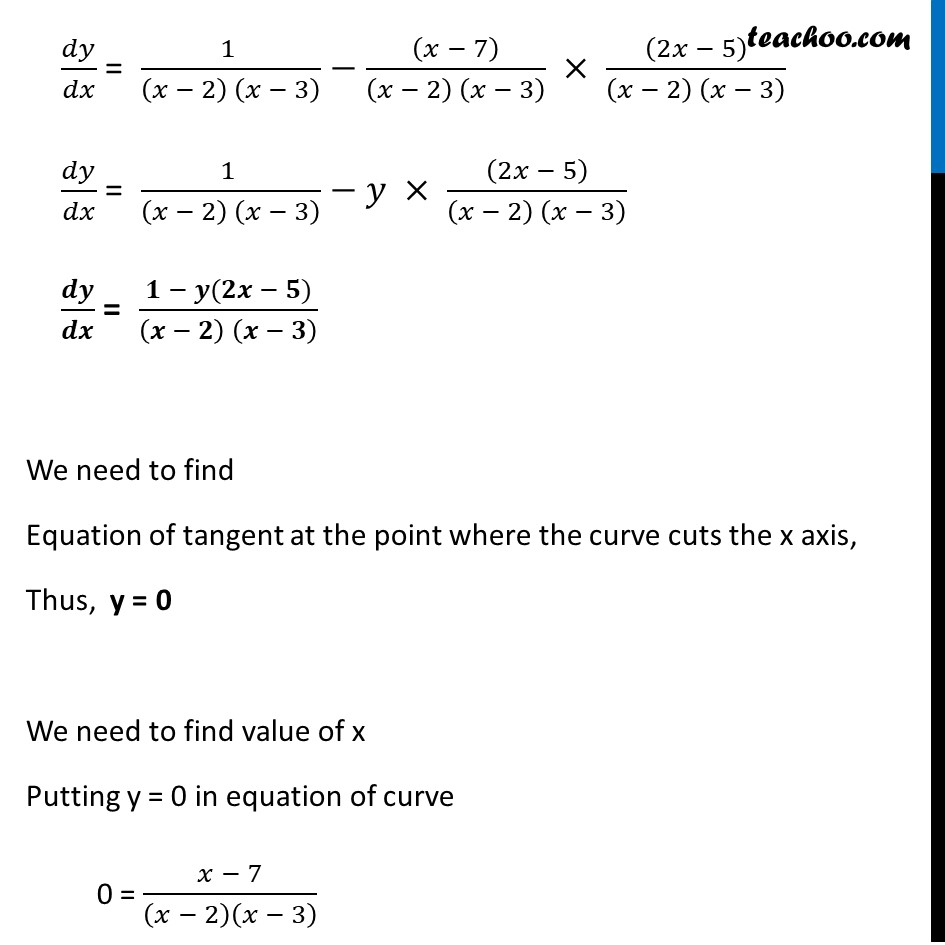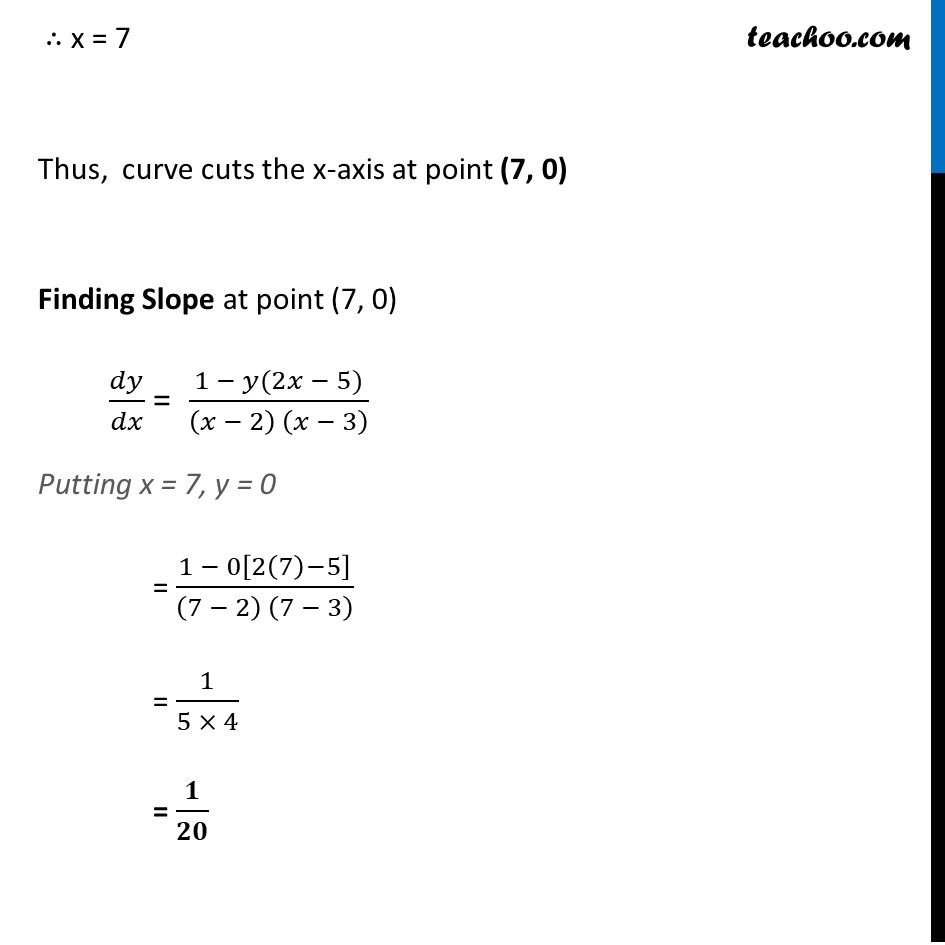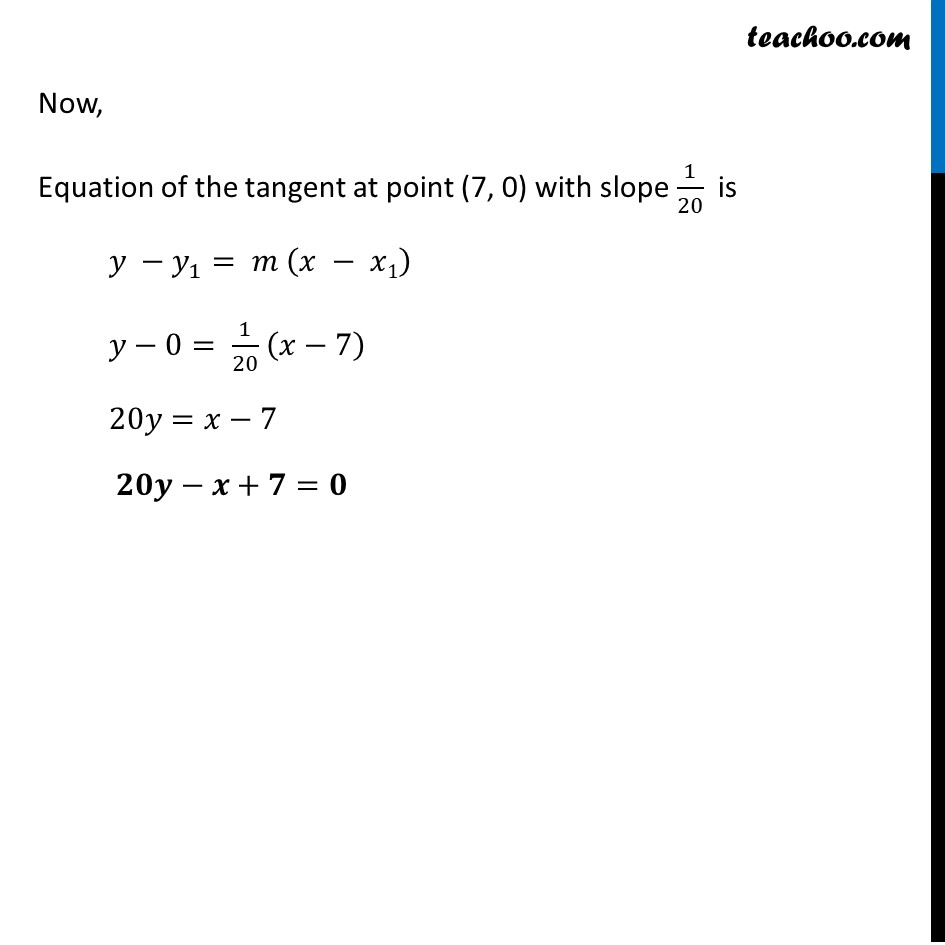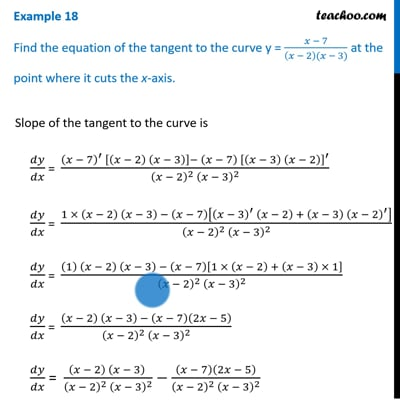This video is only available for Teachoo black users

Learn in your speed, with individual attention - Teachoo Maths 1-on-1 Class

### Transcript

Question 5 Find the equation of the tangent to the curve y = (𝑥 − 7)/((𝑥 − 2)(𝑥 − 3)) at the point where it cuts the x-axis.Slope of the tangent to the curve is 𝑑𝑦/𝑑𝑥 = ((𝑥 − 7)^′ [(𝑥 − 2) (𝑥 − 3)]− (𝑥 − 7) [(𝑥 − 3) (𝑥 − 2)]^′)/((𝑥 − 2)^2 (𝑥 − 3)^2 ) 𝑑𝑦/𝑑𝑥 = (1 × (𝑥 − 2) (𝑥 − 3) − (𝑥 − 7)[(𝑥 − 3)^′ (𝑥 − 2) + (𝑥 − 3) (𝑥 − 2)^′ ])/((𝑥 − 2)^2 (𝑥 − 3)^2 ) 𝑑𝑦/𝑑𝑥 = ((1) (𝑥 − 2) (𝑥 − 3) − (𝑥 − 7)[1 × (𝑥 − 2) + (𝑥 − 3) × 1])/((𝑥 − 2)^2 (𝑥 − 3)^2 ) 𝑑𝑦/𝑑𝑥 = ((𝑥 − 2) (𝑥 − 3) − (𝑥 − 7)(2𝑥 − 5))/((𝑥 − 2)^2 (𝑥 − 3)^2 ) 𝑑𝑦/𝑑𝑥 = ((𝑥 − 2) (𝑥 − 3) )/((𝑥 − 2)^2 (𝑥 − 3)^2 )−(𝑥 − 7)(2𝑥 − 5)/((𝑥 − 2)^2 (𝑥 − 3)^2 ) 𝑑𝑦/𝑑𝑥 = (1 )/((𝑥 − 2) (𝑥 − 3) )−((𝑥 − 7))/((𝑥 − 2) (𝑥 − 3) ) × ((2𝑥 − 5))/((𝑥 − 2) (𝑥 − 3) ) 𝑑𝑦/𝑑𝑥 = (1 )/((𝑥 − 2) (𝑥 − 3) )−𝑦 × ((2𝑥 − 5))/((𝑥 − 2) (𝑥 − 3) ) 𝒅𝒚/𝒅𝒙 = (𝟏 − 𝒚(𝟐𝒙 − 𝟓))/((𝒙 − 𝟐) (𝒙 − 𝟑) ) We need to find Equation of tangent at the point where the curve cuts the x axis, Thus, y = 0 We need to find value of x Putting y = 0 in equation of curve 0 = (𝑥 − 7)/(𝑥 − 2)(𝑥 − 3) ∴ x = 7 Thus, curve cuts the x-axis at point (7, 0) Finding Slope at point (7, 0) 𝑑𝑦/𝑑𝑥 = (1 − 𝑦(2𝑥 − 5))/((𝑥 − 2) (𝑥 − 3) ) Putting x = 7, y = 0 = (1 − 0[2(7)−5])/((7 − 2) (7 − 3) ) = 1/(5 × 4) = 𝟏/𝟐𝟎 Now, Equation of the tangent at point (7, 0) with slope 1/20 is 𝑦 −𝑦_1= 𝑚 (𝑥 − 𝑥1) 𝑦−0= 1/20 (𝑥−7) 20𝑦=𝑥−7 𝟐𝟎𝒚−𝒙+𝟕=𝟎